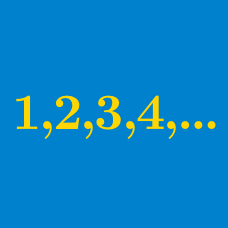Algebra

Arithmetic Progressions: Level 2 Challenges

If two numbers have arithmetic mean 2700 and harmonic mean 75, then find their geometric mean.


Note:

• The arithmetic mean of two numbers $a$ and $b$ is $\frac{a+b}2$.
• The harmonic mean of two numbers $a$ and $b$ is $\frac2{\frac1{a} + \frac1{b}}$.

$1 + 2 - 3 - 4 + 5 + 6 - 7 - 8 + \ldots + 301 + 302 = ?$

Clarification: The sum keeps alternating between two distinct positive numbers and two distinct negative numbers.

Real numbers $a_1,a_2,\ldots,a_{99}$ form an arithmetic progression.

Suppose that $a_2+a_5+a_8+\cdots+a_{98}=205.$ Find the value of $\displaystyle \sum_{k=1}^{99} a_k$.

$a_1,a_2,a_3,\ldots, a_{98}$ are terms in an arithmetic progression with common difference 1 such that their sum is 137.

What is the sum of the even terms of this progression? That is, what is the value of $a_2+a_4+a_6+\cdots+a_{98}$?

$54+51+48+45+ \cdots$

You are given the sum of an arithmetic progression of a finite number of terms, as shown above.

What is the minimum number of terms used to make a total value of 513?

×